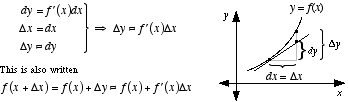index: click on a letter A B C D E F G H I J K L M N O P Q R S T U V W X Y Z A to Z index index: subject areas numbers & symbols sets, logic, proofs geometry algebra trigonometry advanced algebra & pre-calculus calculus advanced topics probability & statistics real world applications multimedia entrieswww.mathwords.com about mathwords website feedback

Approximation by Differentials

A method for approximating the value of a function near a known value. The method uses the tangent line at the known value of the function to approximate the function's graph. In this method Δx and Δy represent the changes in x and y for the function, and dx and dy represent the changes in x and y for the tangent line.Example: Approximate $$\sqrt {10}$$ by differentials. Solution: $$\sqrt {10}$$ is near $$\sqrt 9$$, so we will use $$f\left( x \right) = \sqrt x$$ with x = 9 and Δx = 1. Note that $$f'\left( x \right) = \frac{1}{{2\sqrt x }}$$. \eqalign{ \sqrt {10} &= f\left( {x + \Delta x} \right)\\ &\approx f\left( x \right) + f'\left( x \right)\Delta x\\ &= \sqrt x + \frac{1}{{2\sqrt x }}\Delta x\\ &= \sqrt 9 + \frac{1}{{2\sqrt 9 }}\left( 1 \right)\\ &= 3\frac{1}{6} } . Thus we see that $$\sqrt {10} \approx 3\frac{1}{6} = 3.166\bar 6$$. This is very close to the correct value of $$\sqrt {10} \approx 3.1623$$.